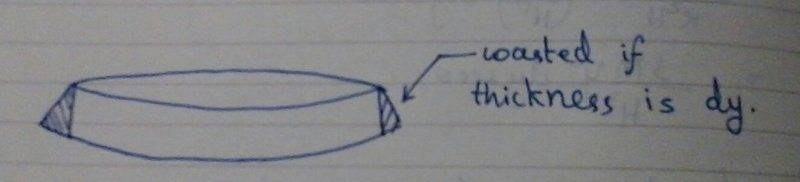# Centre of mass of solid cone

## Homework Statement

Find the centre of mass of solid cone.

## Homework Equations

$$y_{cm}=\frac{1}{M}\int_0^Hydm$$

## The Attempt at a Solution

First I took thin disks. I got the answer when I assumed its thickness to be dy but then dysecθ would be more accurate if half angle of cone is θ since dysecθ gives the length of the slanted part, while dy would give a cylinder.Now I get wrong answer.

ehild
Homework Helper

## Homework Statement

Find the centre of mass of solid cone.

## Homework Equations

$$y_{cm}=\frac{1}{M}\int_0^Hydm$$

## The Attempt at a Solution

First I took thin disks. I got the answer when I assumed its thickness to be dy but then dysecθ would be more accurate if half angle of cone is θ since dysecθ gives the length of the slanted part, while dy would give a cylinder.
But the volume of a truncated cone is (h pi/3 )(r12+r1r2+r22) where r1 and r2 are the radii of the base plates and h is the height. In case of the volume element, r1≈r2=r and h=dy, so the volume element is that of a disk with radius r and height dy.

Also, you have to use density times Ady instead of dm in the integral.

Last edited:
But the volume of a truncated cone is (h pi/3 )(r12+r1r2+r22) where r1 and r2 are the radii of the base plates and h is the height. In case of the volume element, r1≈r2=r and h=dy, so the volume element is that of a disk with radius r and height dy.

Also, you have to use density times dy instead of dm in the integral.
OK. But whats wrong in dysecθ?

ehild
Homework Helper
Nothing is wrong with it if you do not use it instead of dy.